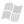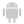Conditions for adding the row containing the custom calculation formulaRecalculating dataCustomizing the calculation row WINDEVWEBDEVWINDEV MobileOthersTableFormulaAdd (Function) In french: TableFormuleAjoute Adds a calculated row to a Table or TreeView Table control by providing custom calculation procedures.Example TableFormulaDeleteAll(TABLE_MyTable)nRow is intnRow = TableFormulaAdd(TABLE_MyTable.COL_Num, "Positive mean", ProcInit, ProcAdd, ProcEnd)// Change the background color of the row for custom calculationCOL_Num[nRow].BackgroundColor = LightRed nCounter is intINTERNAL PROCÉDURE ProcInit() nCounter = 0 RESULT 0END INTERNAL PROCÉDURE ProcAdd(Accumulator, ColValue) // Ignores the negative numbers or NULL IF (ColValue <= 0) RESULT Accumulator nCounter++ RESULT Accumulator + ColValueENDINTERNAL PROCÉDURE ProcEnd(Accumulator) IF nCounter = 0 THEN RESULT 0 // Calculate the mean RESULT Accumulator/nCounterEND nRow2 is intnRow2 = TableFormulaAdd(TABLE_TableControl.COL_Time, "Average duration", Null, ... AverageDuration_Iteration, AverageDuration_End) INTERNAL PROCÉDURE AverageDuration_Iteration(Accumulator, ColValue) RESULT Accumulator + [TAB] + ColValueEND INTERNAL PROCÉDURE AverageDuration_End(Accumulator) nSum is 8-byte int nNbValid is int FOR EACH STRING sValue OF Accumulator SEPARATED BY TAB IF ValidTime(sValue) THEN nSum += TimeToInteger(sValue) nNbValid++ END END RESULT TimeToString(IntegerToTime(nSum/nNbValid), "HH:MM:SS")END Syntax = TableFormulaAdd( , , , , ) : IntegerIndex of the row containing the formula. : Control nameName of column into which the formula will be added.If this parameter corresponds to an empty string (""), the column to which the current process belongs will be used. : Character stringCaption of the additional row where the calculation will be displayed. If this caption does not exist, the row will be created. If this caption exists but not for the specified column, the calculation is displayed in the existing row but for the specified column. If this caption exists for the specified column, a WLanguage error occurs. : Procedure name or NULLName of the WLanguage procedure ("callback") called to initialize the formula. This procedure has the following format: PROCEDURE ()// your codeRESULT where is the return value for the first iteration of the calculation.NULL if the formula requires no initialization process. : Procedure name or NULLName of the WLanguage procedure ("callback") called for each iteration of the formula (each row of the Table control). This procedure has read-only access to the columns of each row. This procedure has the following format: PROCEDURE (, )// Called for each row in the Table control// Your codeRESULT where: is the value coming from the previous calculation (initialization or previous iteration). is the value of the current column used to calculate this iteration. is the new value to return for the next iteration or at the end of calculation.NULL if the formula requires no iteration process. : Character stringName of the WLanguage procedure ("callback") called to end the formula. This procedure has the following format: PROCEDURE  ()// your codeRESULT where: is the value coming from the previous calculation (initialization or previous iteration). is the return value that corresponds to the end value of the calculation.NULL if the formula requires no ending process. Remarks Conditions for adding the row containing the custom calculation formulaIf the calculation named does not exist in the Table control, a new calculation row is added below the Table control (after the existing calculations). If a calculation named was already defined for another column, the calculation is displayed for the specified column in the existing calculation row.If a calculation named was already defined for the same column, a WLanguage error occurs.Only 5 custom calculation rows can be added. Remark: This function can be used: on the columns of a Table or TreeView Table control based on a data file. on the columns of a Table or TreeView Table control populated programmatically. Recalculating dataThe custom calculation rows are automatically recalculated as soon as the content of the Table control changes.Tip: The iteration procedure is called for each row: we advise you not to perform slow calculations (avoid the accesses to the database for example).Customizing the calculation rowThe following syntax is used to customize the added row (caption, color, font, height, ...): [Row number]. = where can correspond to one of the properties that can be used on a column of a Table control. For more details, see Programming custom calculations in Table controls. Business / UI classification: UI CodeComponent: wd280obj.dll Minimum version required Version 22 This page is also available for…